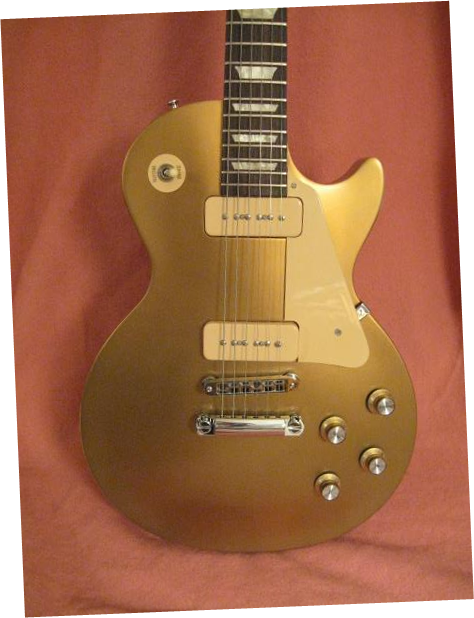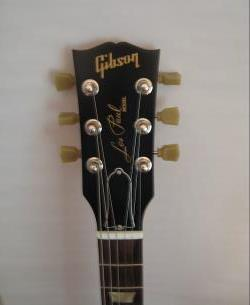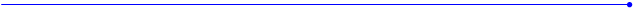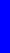The Nealon Equationy  =  (A*sin(B*x+C) + D*sin(E*x+F) + G*sin(H*x+I) + J*sin(K*x+L)) *  (M + N*x + O*x^2 + P*x^3 + Q*x^4) * atan(R*x) * atan(S*(BL-x))Gibson Les Paul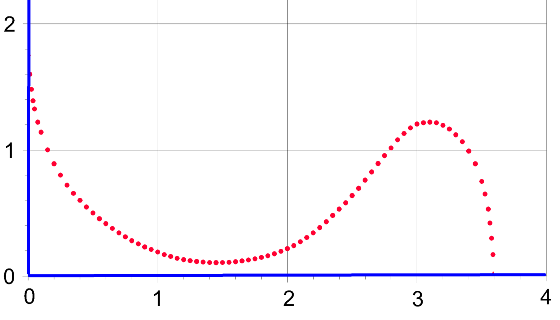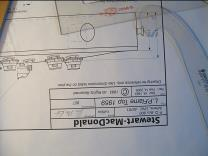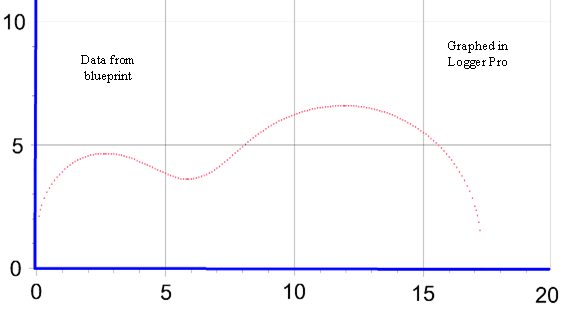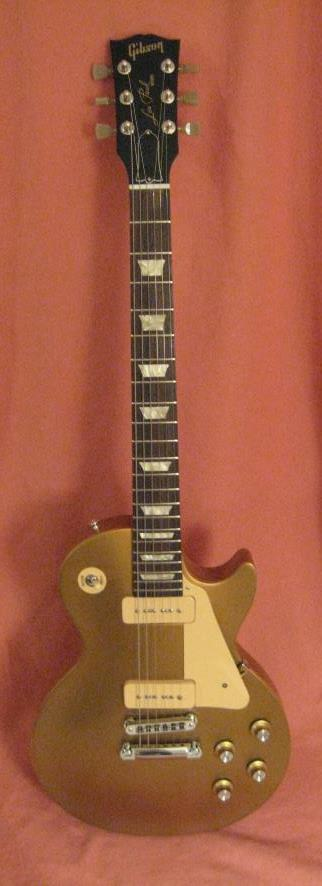y = (A*sin(B*x+C)+D*sin(E*x+F)+G*sin(H*x+I)+J*sin(K*x+L))*(M+N*x+O*x^2+P*x^3+Q*x^4)*atan(R*x)*atan(S*(17.275-x))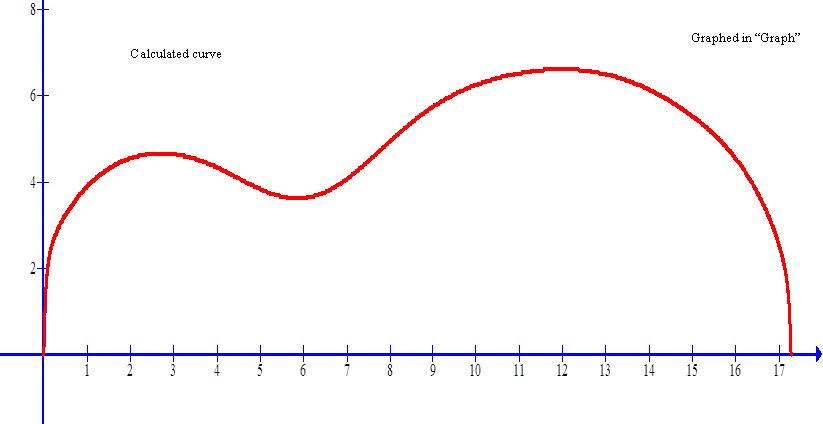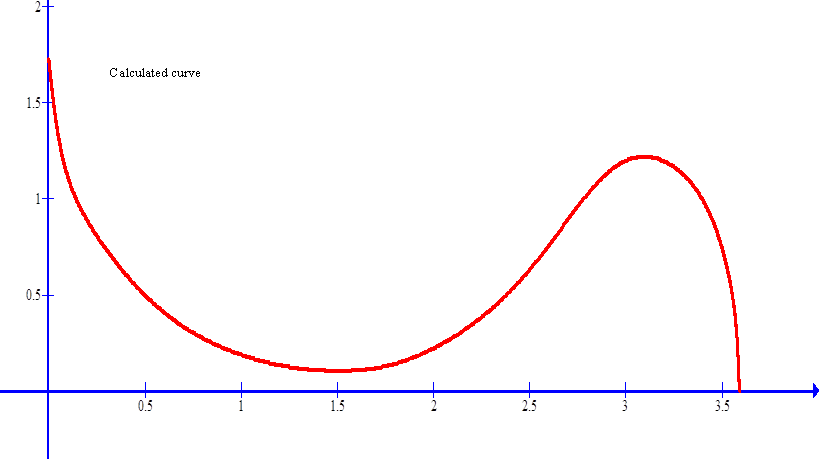The two curves from the graphs put together.
 The drawing is of a 1959 flame top.
 y = (A*sin(B*x+C)+D*sin(E*x+F)+G*sin(H*x+I)+J*sin(K*x+L))*(M+N*x+O*x^2+P*x^3+Q*x^4)*(3-atan(R*x))*atan(S*(3.59-x))
 Cutaway A modified equation is required for the cutaway.  The first arctan term is subtracted from 3.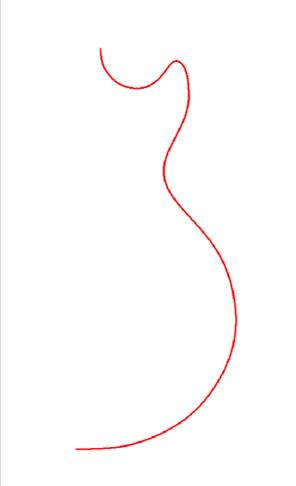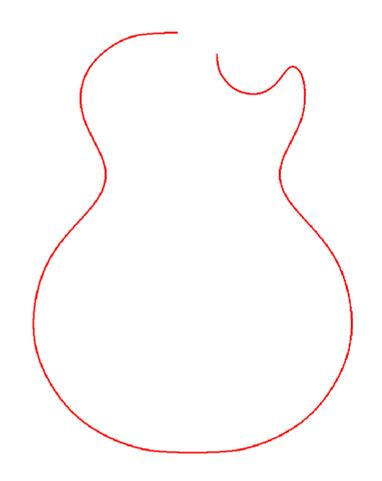Now the body twice, one mirror image of the other and the cutaway.  All of this is done with the curves produced by the mathematical equation.  I suppose it also could be done with the point series of data taken from the instrument, but what’s the fun in that?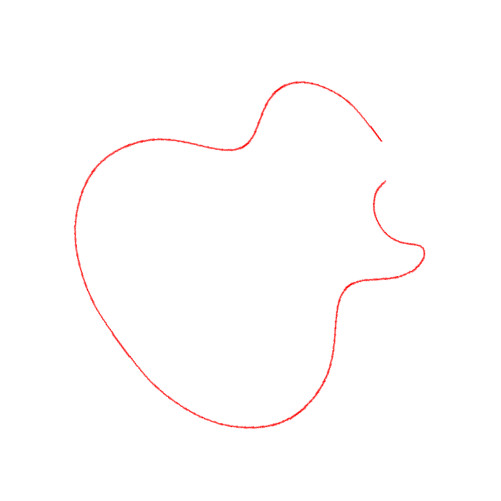Length around the perimeter of the bass side of the Les Paul.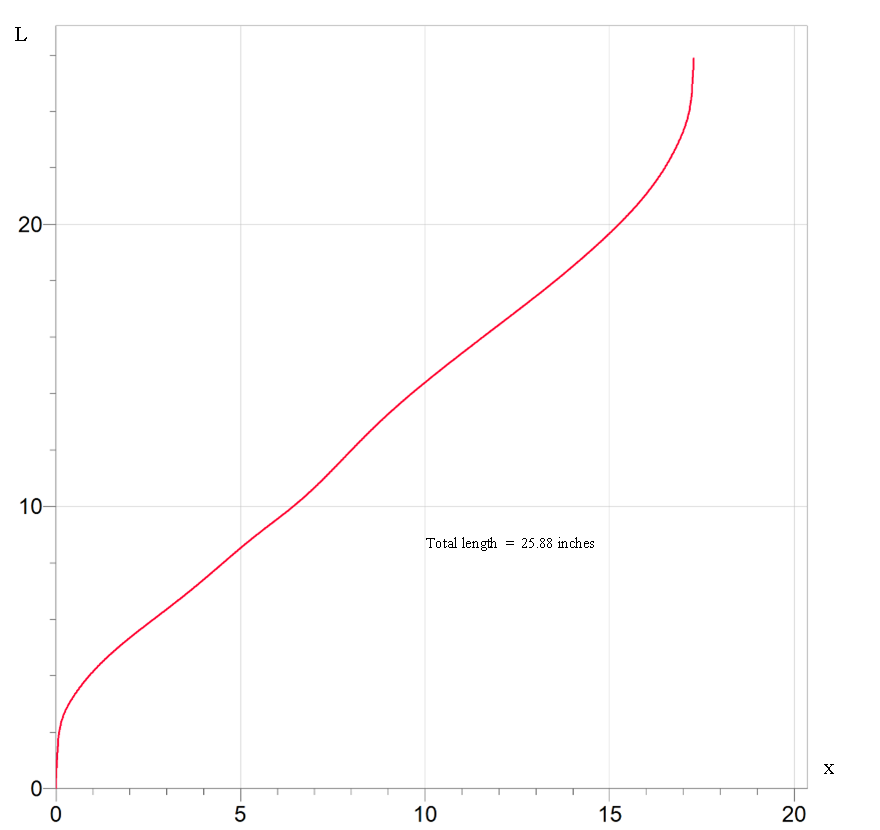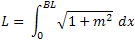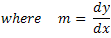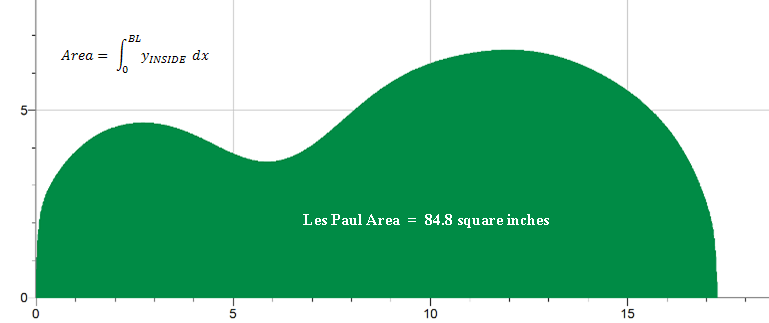Les Paul Body Outline A 0.3661125 B -0.6542102 C -3.428853 D 0.04257652 E -1.426924 F 0.9901923 G 4.458389 H 0.07430593 I 1.892568 J 1.918956 K 0.3106034 L -2.663953 M 0.3029042 N 0.2545602 O -0.0215465 P -0.0007298772 Q 8.45777E-05 R 24.62258 S 23.2647 BL 17.275 A B C D E F G H I J K L M N O P Q R S BL 0.3661125 -0.6542102 -3.428853 0.04257652 -1.426924 0.9901923 4.458389 0.07430593 1.892568 1.918956 0.3106034 -2.663953 0.3029042 0.2545602 -0.0215465 -0.0007298772 8.45777E-05 24.62258 23.2647 17.275 A = 0.3661125 B = -0.6542102 C = -3.428853 D = 0.04257652 E = -1.426924 F = 0.9901923 G = 4.458389 H = 0.07430593 I = 1.892568 J = 1.918956 K = 0.3106034 L = -2.663953 M = 0.3029042 N = 0.2545602 O = -0.0215465 P = -0.0007298772 Q = 8.45777E-05 R = 24.62258 S = 23.2647 BL = 17.275
 Les Paul Cutaway A 0.002098982 B -6.793013 C 1.691257 D 3.204554 E -0.8213864 F 1.118254 G 2.366427 H -0.2342140 I 0.29424849 J 4.349017 K -0.7233598 L 4.076135 M 5.072948 N -11.91285 O 12.03647 P -5.864441 Q 1.146872 R 16.64988 S 98.20209 BL 3.59 A B C D E F G H I J K L M N O P Q R S BL 0.002098982 -6.793013 1.691257 3.204554 -0.8213864 1.118254 2.366427 -0.2342140 0.29424849 4.349017 -0.7233598 4.076135 5.072948 -11.91285 12.03647 -5.864441 1.146872 16.64988 98.20209 3.59 A = 0.002098982 B = -6.793013 C = 1.691257 D = 3.204554 E = -0.8213864 F = 1.118254 G = 2.366427 H = -0.2342140 I = 0.29424849 J = 4.349017 K = -0.7233598 L = 4.076135 M = 5.072948 N = -11.91285 O = 12.03647 P = -5.864441 Q = 1.146872 R = 16.64988 S = 98.20209 BL = 3.59